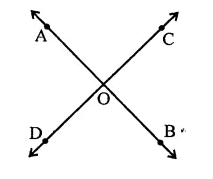# RD Sharma Class 9 Solutions Chapter 10 Congruent Triangles VSAQS

## RD Sharma Class 9 Solutions Chapter 10 Congruent Triangles VSAQS

These Solutions are part of RD Sharma Class 9 Solutions. Here we have given RD Sharma Class 9 Solutions Chapter 10 Congruent Triangles VSAQS

Other Exercises

Question 1.
Define complementary angles.
Solution:
Two angles whose sum is 90°, are called complementary angles.

Question 2.
Define supplementary angles.
Solution:
Two angles whose sum is 180°, are called supplementary angles.

Question 3.
Solution:
Two angles which have common vertex and one arm common are called adjacent angles.

Question 4.
The complement of an acute angles is…….
Solution:
The complement of an acute angles is an acute angle.

Question 5.
The supplement of an acute angles is………
Solution:
The supplement of an acute angles is a obtuse angle.

Question 6.
The supplement of a right angle is…….
Solution:
The supplement of a right angle is a right angle.

Question 7.
Write the complement of an angle of measure x°.
Solution:
The complement of x° is (90° – x)°

Question 8.
Write the supplement of an angle of measure 2y°.
Solution:
The supplement of 2y° is (180° – 2y)°

Question 9.
If a wheel has six spokes equally spaced, then find the measure of the angle between two adjacent spokes.
Solution:
Total measure of angle around a point = 360°
Number of spokes = 6
∴ Angle between the two adjacent spokes = $$\frac { { 360 }^{ \circ } }{ 6 }$$ = 60°

Question 10.
An angle is equal to its supplement. Determine its measure.
Solution:
Let required angle = x°
Then its supplement angle = 180° – x
x = 180° – x
⇒ x + x = 180°
⇒  2x = 180° ⇒  x = $$\frac { { 180 }^{ \circ } }{ 2 }$$ = 90°
∴ Required angle = 90°

Question 11.
An angle is equal to five times its complement. Determine its measure.
Solution:
Let required measure of angle = x°
∴  Its complement angle = 90° – x
∴  x = 5(90° – x)
⇒  x = 450° – 5x
⇒  x + 5x = 450°
⇒  6x = 450°
⇒ x = $$\frac { { 450 }^{ \circ } }{ 6 }$$ = 75°
∴ Required angle = 75°

Question 12.
How many pairs of adjacent angles are formed when two lines intersect in a point?
Solution:
If two lines AB and CD intersect at a point O, then pairs of two adjacent angles are, ∠AOC and ∠COB, ∠COB and ∠BOD, ∠BOD and DOA, ∠DOA and ∠ZAOC
i.e, 4 pairsHope given RD Sharma Class 9 Solutions Chapter 10 Congruent Triangles VSAQS are helpful to complete your math homework.

If you have any doubts, please comment below. Learn Insta try to provide online math tutoring for you.